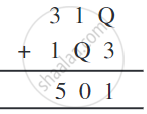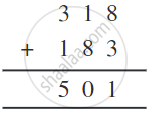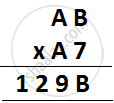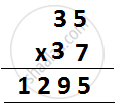# Letters for Digit:

Here we have puzzles in which letters take the place of digits in an arithmetic ‘sum’, and the problem is to find out which letter represents which digit; so it is like cracking a code. Here we stick to problems of addition and multiplication.

Here are two rules we follow while doing such puzzles.

1. Each letter in the puzzle must stand for just one digit. Each digit must be represented by just one letter.
2. The first digit of a number cannot be zero. Thus, we write the number “fifty-three” as 53, and not as 053, or 0053.

1) Find Q in the addition.Solution:
There is just one letter Q whose value we have to find.
Study the addition in the one's column: from Q + 3, we get ‘1’, that is, a number whose one's digit is 1.
For this to happen, the digit Q should be 8. So the puzzle can be solved as shown below.That is, Q = 8

## 2) Puzzles with multiplication:

Find the value of A and B in the below multiplication.B × 7 = B, it is possible if B = 0 or B = 5

If A = 1 and B = 0, AB × A7 = 10 × 17 = 170

If A = 1 and B = 5, AB × A7 = 15 × 17 = 255

If A = 2 and B = 0, AB × A7 = 20 × 27 = 540

If A = 2 and B= 5, AB × A7 = 25 × 27 = 675

If A = 3 and B= 0, AB × A7 = 30 × 37 = 1110

If A = 3 and B = 5, AB × A7 = 35 × 37 = 1295#### Example

Find A and B in the addition.
 A + A + A B A
The sum of three A’s is a number whose one's digit is A.
The sum of two A’s must be a number whose one's digit is 0.
This happens only for A = 0 and A = 5.
If A = 0, then the sum is 0 + 0 + 0 = 0, which makes B = 0 too.
We do not want this, so we reject this possibility.
So, A = 5.
Therefore, the puzzle is solved as shown below.
 5 + 5 + 5 1 5
That is, A = 5 and B = 1.
If you would like to contribute notes or other learning material, please submit them using the button below.

### Shaalaa.com

Letters for Digits | [00:37:54]
S
0%